# Arduino_Motor_Shield__L298N___SKU_DRI0009_-DFRobot

## Introduction

This 2x2A DC Motor Shield for Arduino allows Arduino to drive two channel DC motors. It uses a L298N chip which deliveries output current up to 2A each channel. The speed control is achieved through conventional PWM which can be obtained from Arduino’s PWM output Pin 5 and 6. The enable/disable function of the motor control is signalled by Arduino Digital Pin 4 and 7.

The Motor shield can be powered directly from Arduino or from external power source. It is strongly encouraged to use external power supply to power the motor shield.

• Logic Control Voltage：5V (From Arduino)
• Motor Driven Voltage：4.8～35V (From Arduino or External Power Source)
• Logic supply current Iss：≤36mA
• Motor Driven current Io：≤2A
• Maximum power consumption：25W（T=75℃）
• PWM, PLL Speed control mode
• Control signal level:

High：2.3V≤Vin≤5V Low：-0.3V≤Vin≤1.5V

### Board Diagram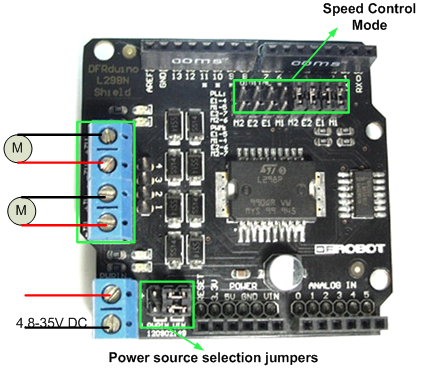Control Mode Selection Jumpers: The shield supports PWM and PLL(Phased Locked Loop) control Modes. The PWM mode uses E1 and E2 to generate PWM signal. The PLL mode uses M1 and M2 to generate phase control signal.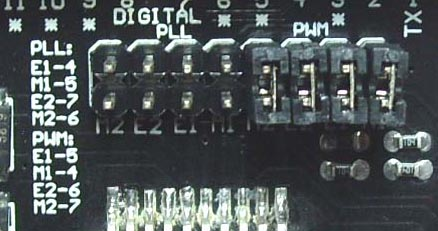Motor Terminal: Two DC motors are connected to blue motor terminals. The male header behide the terminals are the same as the motor terminals.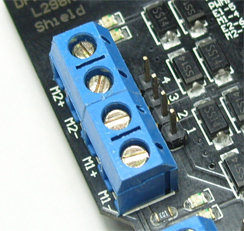PWRIN： The motors can be powered by external power supply when the motor current exceeds the limits provided from the Arduino. The swith between external and Arduino power is implemented by two jumpers.

• PWRIN: External Power

• VIN: Arduino Power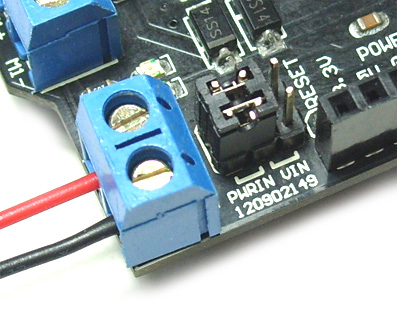NOTE: When the motor shield is powered by external power source, make sure the external power source and Arduino have the same GND.

Control Signal Truth Table：

E1 M1 E2 M2
L X Motor 1 Disabled L X Motor 2 Disabled
H H Motor 1 Backward H H Motor 2 Backward
PWM X PWM Speed control PWM X PWM Speed control

Note：H is High level; L is Low level; PWM is Pulse Width Modulation signal; X is any voltage level

### Pin Allocation

Pin Function
Digital 4 Motor 1 Direction control
Digital 5 Motor 1 PWM control
Digital 6 Motor 2 PWM control
Digital 7 Motor 2 Direction control

"PWM Mode"

Pin Function
Digital 4 Motor 1 Enable control
Digital 5 Motor 1 Direction control
Digital 6 Motor 2 Direction control
Digital 7 Motor 2 Enable control

"PLL Mode"

### Sample Code

#### PWM Speed Control

``````//Arduino PWM Speed Control：
int E1 = 5;
int M1 = 4;
int E2 = 6;
int M2 = 7;

void setup()
{
pinMode(M1, OUTPUT);
pinMode(M2, OUTPUT);
}

void loop()
{
int value;
for(value = 0 ; value <= 255; value+=5)
{
digitalWrite(M1,HIGH);
digitalWrite(M2, HIGH);
analogWrite(E1, value);   //PWM Speed Control
analogWrite(E2, value);   //PWM Speed Control
delay(30);
}
}``````

#### PLL Speed Control

``````//Arduino PLL Speed Control：
int E1 = 4;
int M1 = 5;
int E2 = 7;
int M2 = 6;

void setup()
{
pinMode(M1, OUTPUT);
pinMode(M2, OUTPUT);
}

void loop()
{
int value;
for(value = 0 ; value <= 255; value+=5)
{
digitalWrite(M1,HIGH);
digitalWrite(M2, HIGH);
analogWrite(E1, value);   //PLL Speed Control
analogWrite(E2, value);   //PLL Speed Control
delay(30);
}
}``````

## More Documents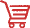Get Arduino Motor Shield (L298N) from DFRobot Store or DFRobot Distributor.

Turn to the Top